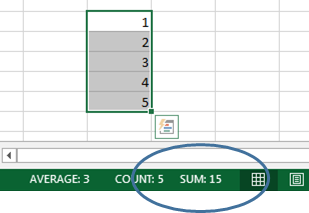Categories

# SUM function in EXCEL

### Way to do SUM in EXCEL

1. With AUTOSUM Function

You will find this in Home Tab Ribbon -> Editing group -> AutoSum

2. With sortcut => “Alt +”

This will automatically give SUM function and tale nearby range for summation3. By typing SUM function with proper Syntax

ex. =SUM(A1:A10)

If range is not in squence, then separate is by comma

ex. =SUM(A1:A10, A15)

4. One more quick way directly by cell reference or values with operator +

ex. =A1+A10  (This will add values of A1 & A10)

or

=1+2+A47  (This will Add 1 & 2 with value of A47 cell)

5. You just cant believe one more way

Select all the values you need to check its SUM, and see on status bar, you will get Answer right there